We want to estimate the probability of success / failure of the dependent variable ($$\Pr(Y=1)$$), given the explanatory variables: $$\Pr(Y=1|X_1,X_2,\cdots, X_n)$$. The explanatory variables can be categorical or continuous - it doesn’t matter.

Logistic regression addresses the following issues:

1. There is a need to bound at $$1$$ since we are estimating a probability, but lines are not naturally bounded. So we transform probability into odds:

$\text{odds}=\frac{\Pr(Y=1)}{1-\Pr(Y=1)}\tag 1$

In addition, this also helps turning a sigmoid curve of a typical cummulative probability distribution (e.g. normal) into a linear relation.

1. Now, when the probability goes down to zero, the odds also tend to zero; yet there is no floor restriction in a line. This problem can be addressed by expressing the linear relation in log scale: $$\log(\text{odds})$$, which will tend to $$-\infty$$ as the odds tend to zero. This is the logit or log-odds function.

The logistic regression model is therefore:

$$\large \color{red}{\log} \left[\color{blue}{\text{odds(p(Y=1))}}\right]=\color{red}{\text{log}}\left(\frac{\hat p\,(Y=1)}{1-\hat p\,(Y=1)}\right) = X\beta = \beta_o + \beta_1 x_1 + \beta_2 x_2 +\cdots+ \beta_p x_p$$

Consequently,

$\large \color{blue}{\text{odds(Y=1)}} = \frac{\Pr\,(Y=1)}{1\,-\,\Pr\,(Y=1)} = e^{\,\beta_0 + \beta_1 x_1 + \cdots + \beta_p x_p} = e^{\,\beta_o}\,e^{\,\beta_1x_1}\,e^{\,\beta_2x_2}\,\cdots\,e^{\,\beta_px_p}$

Therefore a unit increase in $$x_1$$ increases the odds $$\large e^{\,\beta_1}$$ times. The factors $$\large e^{\,\beta_i}$$ are the ODDS RATIO’s. On the other hand, $$\beta_i$$ (the coefficients) are the LOG ODDS-RATIO: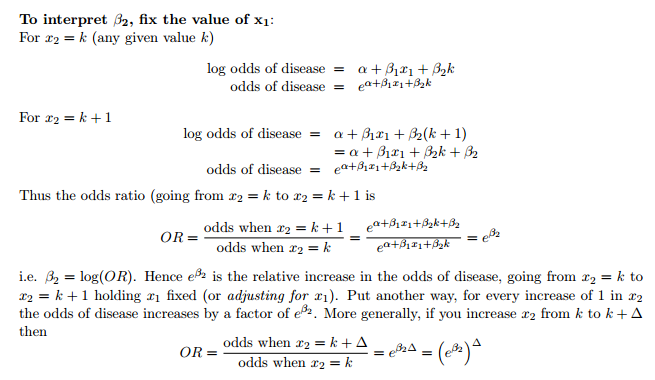For CATEGORICAL VARIABLES, the ODDS RATIO denote how much the presence or absence of a factor variable increases the odds of a positive dependent variable.

For CONTINOUS VARIABLES the odds ratio tell us how much the odds increase multiplicatively with a one-unit change in the independent variable. To calculate the difference in odds you raise the OR to the power of the difference between the observations.

To get the exponentiated coefficients and their confidence intervals in R, we use cbind to bind the coefficients and confidence intervals column-wise:

exp(cbind(OR = coef(mylogit), confint(mylogit)))

See here.

#### Translation into probabilities:

From equation $$(1):$$

\begin{align} \Pr(Y = 1) &= (1-\Pr(Y=1))\times\,e^{\,\beta_0 + \beta_1 x_1 + \cdots + \beta_p x_p}\\[2ex] e^{\,\beta_0 + \beta_1 x_1 + \cdots + \beta_p x_p}&= \Pr(Y = 1)\,\times (1 \,+\,e^{\,\beta_0 + \beta_1 x_1 + \cdots + \beta_p x_p}) \\[2ex] \color{green}{\Pr(Y = 1)} = \frac{\color{blue}{\text{odds(Y=1)}}}{1\,+\,\color{blue}{\text{odds(Y=1)}}}&=\frac{e^{\,\beta_0 + \beta_1 x_1 + \cdots + \beta_p x_p}}{1 \,+\,e^{\,\beta_0 + \beta_1 x_1 + \cdots + \beta_p x_p}}=\frac{1}{1 \,+\,e^{-(\,\beta_0 + \beta_1 x_1 + \cdots + \beta_p x_p)}} \end{align}

####INTERPRETATION OF LOGISTIC REGRESSION RESULTS:

QUESTION:

(Initially posted here)

I ran a linear regression of acceptance into college against SAT scores and family / ethnic background. The data are fictional. The question focuses in the gathering and interpretation of odds ratios when leaving the SAT scores aside for simplicity.

The variables are Accepted (0 or 1) and Background (“red” or “blue”). I set up the data so that people of “red” background were more likely to get in:

fit <- glm(Accepted ~ Background, data=dat, family="binomial")
exp(cbind(Odds_Ratio_RedvBlue = coef(fit), confint(fit)))

Odds_Ratio_RedvBlue             2.5 %       97.5 %
(Intercept)             0.7088608                     0.5553459   0.9017961
Backgroundred           2.4480042                     1.7397640   3.4595454

Questions:

1. Is 0.7 the odd ratio of a person of “blue” background being accepted? I’m asking this because I also get 0.7 for “Backgroundblue” if instead I run the following code:

 fit <- glm(Accepted~Background - 1, data=dat, family="binomial")
exp(cbind(OR=coef(fit), confint(fit)))
2. Shouldn’t the odds ratio of “red” being accepted ($$\rm Accepted/Red:Accepted/Blue$$) just the reciprocal: ($$\rm OddsBlue = 1 / OddsRed$$)?

I’ve been working on answering my question by calculating manually the odds and odds ratios:

Acceptance   blue            red            Grand Total
0              158           102                260
1              112           177                289
Total          270           279                549

So the Odds Ratio of getting into the school of Red over Blue is:

$\frac{\rm Odds\ Accept\ If\ Red}{\rm Odds\ Acccept\ If\ Blue} = \frac{^{177}/_{102}}{^{112}/_{158}} = \frac {1.7353}{0.7089} = 2.448$

And this is the Backgroundredreturn of:

fit <- glm(Accepted~Background, data=dat, family="binomial")
exp(cbind(Odds_and_OR=coef(fit), confint(fit)))

Odds_and_OR                         2.5 %      97.5 %
(Intercept)             0.7088608                     0.5553459   0.9017961
Backgroundred           2.4480042                     1.7397640   3.4595454

At the same time, the (Intercept)corresponds to the numerator of the odds ratio, which is exactly the odds of getting in being of ‘blue’ family background: $$112/158 = 0.7089$$.

fit2 <- glm(Accepted~Background-1, data=dat, family="binomial")
exp(cbind(Odds=coef(fit2), confint(fit2)))

Odds            2.5 %      97.5 %
Backgroundblue     0.7088608        0.5553459   0.9017961
Backgroundred      1.7352941        1.3632702   2.2206569

The returns are precisely the odds of getting in being ‘blue’: Backgroundblue (0.7089) and the odds of being accepted being ‘red’: Backgroundred (1.7353). No Odds Ratio there. Therefore the two return values are not expected to be reciprocal.

Finally, How to read the results if there are 3 factors in the categorical regressor?

Same manual versus [R] calculation:

I created a different fictitious data set with the same premise, but this time there were three ethnic backgrounds: “red”, “blue” and “orange”, and ran the same sequence:

First, the contingency table:

Acceptance  blue    orange  red   Total
0             86        65  130     281
1             64        42  162     268
Total        150       107  292     549

And calculated the Odds of getting in for each ethnic group:

• Odds Accept If Red = 1.246154;
• Odds Accept If Blue = 0.744186;
• Odds Accept If Orange = 0.646154

As well as the different Odds Ratios:

• OR red v blue = 1.674519;
• OR red v orange = 1.928571;
• OR blue v red = 0.597186;
• OR blue v orange = 1.151717;
• OR orange v red = 0.518519; and
• OR orange v blue = 0.868269

And proceeded with the now routine logistic regression followed by exponentiation of coefficients:

fit <- glm(Accepted~Background, data=dat, family="binomial")
exp(cbind(ODDS=coef(fit), confint(fit)))

ODDS     2.5 %   97.5 %
(Intercept)      0.7441860 0.5367042 1.026588
Backgroundorange 0.8682692 0.5223358 1.437108
Backgroundred    1.6745192 1.1271430 2.497853

Yielding the odds of getting in for “blues” as the (Intercept), and the Odds Ratios of Orange versus Blue in Backgroundorange, and the OR of Red v Blue in Backgroundred .

On the other hand, the regression without intercept predictably returned just the three independent odds:

fit2 <- glm(Accepted~Background-1, data=dat, family="binomial")
exp(cbind(ODDS=coef(fit2), confint(fit2)))

ODDS     2.5 %    97.5 %
Backgroundblue   0.7441860 0.5367042 1.0265875
Backgroundorange 0.6461538 0.4354366 0.9484999
Backgroundred    1.2461538 0.9900426 1.5715814

####CATEGORICAL INDEPENDENT VARIABLES:

Here is an example extracted form this site:

# Define an explanatory variable with two levels:
parentsmoke <- as.factor(c(1,0))
# create response vector:
response <- cbind(yes=c(816,188), no=c(3203,1168))
response
##      yes   no
## [1,] 816 3203
## [2,] 188 1168
smoke.logistic <- glm(response~parentsmoke, family=binomial(link=logit))
smoke.logistic
##
## Call:  glm(formula = response ~ parentsmoke, family = binomial(link = logit))
##
## Coefficients:
##  (Intercept)  parentsmoke1
##      -1.8266        0.4592
##
## Degrees of Freedom: 1 Total (i.e. Null);  0 Residual
## Null Deviance:       29.12
## Residual Deviance: 4.365e-13     AIC: 19.24
summary(smoke.logistic)
##
## Call:
## glm(formula = response ~ parentsmoke, family = binomial(link = logit))
##
## Deviance Residuals:
##   0  0
##
## Coefficients:
##              Estimate Std. Error z value Pr(>|z|)
## (Intercept)  -1.82661    0.07858 -23.244  < 2e-16 ***
## parentsmoke1  0.45918    0.08782   5.228 1.71e-07 ***
## ---
## Signif. codes:  0 '***' 0.001 '**' 0.01 '*' 0.05 '.' 0.1 ' ' 1
##
## (Dispersion parameter for binomial family taken to be 1)
##
##     Null deviance: 2.9121e+01  on 1  degrees of freedom
## Residual deviance: 4.3654e-13  on 0  degrees of freedom
## AIC: 19.242
##
## Number of Fisher Scoring iterations: 2

This information gives us the fitted model:

$\text{logit}(\hat\pi_i)=\log\frac{\hat\pi_i}{1-\hat\pi_i}=\hat\beta_0+\hat\beta_1X_i=-1.837+0.459\,(\text{parents smoke = 1})$ where parentsmoke is a dummy variable ((e.g. design variable) that takes value $$1$$ if at least one parents is smoking and $$0$$ if neither is smoking.

parent smoking = 0 is the baseline. We are modeling here probability of “children smoking”. Estimated $$\beta_0=1.827$$ with a standard error of $$0.078$$ is significant and it says that log-odds of a child smoking versus not smoking if neither parents is smoking (the baseline level) is $$-1.827$$ and it’s statistically significant.

Estimated $$\beta_1=0.459$$ with a standard error of $$0.088$$ is significant and it says that log-odds-ratio of a child smoking versus not smoking if at least one parent is smoking versus neither parents is smoking (the baseline level) is $$0.459$$ and it’s statistically significant. $$\exp(0.459)=1.58$$ are the estimated odds-ratios.

Why not just ran a Chi square test:

prop.test(response, correct = F)
##
##  2-sample test for equality of proportions without continuity
##  correction
##
## data:  response
## X-squared = 27.677, df = 1, p-value = 1.434e-07
## alternative hypothesis: two.sided
## 95 percent confidence interval:
##  0.04218944 0.08659558
## sample estimates:
##    prop 1    prop 2
## 0.2030356 0.1386431

It does detect differences in proportions but it doesn’t address:

• Modeling the “risk” of student smoking as a function of parents’ smoking behavior.
• Describing the differences between student smokers and nonsmokers as a function of parents smoking behavior via descriptive discriminate analyses. -Predicting the probabilities that individuals fall into two categories of the binary response as a function of some explanatory variables, e.g. what is the probability that a student is a smoker given that neither of his/her parents smokes.
• Predicting that a student is a smoker given that neither of his/her parents smokes, i.e. probabilities that individuals fall into two categories of the binary response as a function of some explanatory variables, we want to classify new students into “smoking” or “nonsmoking” group depending on parents smoking behavior. we want to develop a social network model, adjust for “bias”, These are just some of the possibilities of logistic regression, which cannot be handled within a framework of goodness-of-fit only.

Here is the same model splitting mothers and fathers as predictors:

kids.smoke <- matrix(c(188,599,816-205,1168,1456,3203-1456), nrow = 3)
dimnames(kids.smoke) = list(Parents = c("None", "Mother", "Father"), Kids = c("Smokers", "Non-smokers"))
kids.smoke
##         Kids
## Parents  Smokers Non-smokers
##   None       188        1168
##   Mother     599        1456
##   Father     611        1747
bad.example <- as.factor(c(0,"1Mother","2Father"))
smoke.logistic
##
## Call:  glm(formula = kids.smoke ~ bad.example, family = binomial(link = logit))
##
## Coefficients:
##            -1.8266              0.9384              0.7760
##
## Degrees of Freedom: 2 Total (i.e. Null);  0 Residual
## Null Deviance:       119.2
## Residual Deviance: -1.06e-12     AIC: 28.77
summary(smoke.logistic)
##
## Call:
##
## Deviance Residuals:
##   0  0  0
##
## Coefficients:
##                    Estimate Std. Error z value Pr(>|z|)
## (Intercept)        -1.82661    0.07858 -23.244   <2e-16 ***
## bad.example1Mother  0.93842    0.09237  10.160   <2e-16 ***
## bad.example2Father  0.77605    0.09157   8.475   <2e-16 ***
## ---
## Signif. codes:  0 '***' 0.001 '**' 0.01 '*' 0.05 '.' 0.1 ' ' 1
##
## (Dispersion parameter for binomial family taken to be 1)
##
##     Null deviance:  1.1915e+02  on 2  degrees of freedom
## Residual deviance: -1.0603e-12  on 0  degrees of freedom
## AIC: 28.768
##
## Number of Fisher Scoring iterations: 2

An example with credit defaults with some calculations extracted from here:

set.seed(123)

gender <- factor(round(runif(100,0,1)),
labels=c("female", "male"))
age <- round(runif(100,20,80))
profession <- factor(round(runif(100,1,4)),
labels=c("student", "worker", "teacher", "self-employed"))

# Let's make males, teachers and self-employed more likely to default:

credit.default <-  round(((as.numeric(gender)/2 + as.numeric(profession)/4)/2) * runif(100,0.5,1))

df <- data.frame(credit.default,gender,age,profession)
head(df)
##   credit.default gender age    profession
## 1              0 female  56        worker
## 2              1   male  40 self-employed
## 3              1 female  49       teacher
## 4              1   male  77       teacher
## 5              1   male  49        worker
## 6              1 female  73 self-employed
catlogis <- glm(credit.default ~ gender+age+profession, df, family=binomial(link="logit"))
summary(catlogis)
##
## Call:
## glm(formula = credit.default ~ gender + age + profession, family = binomial(link = "logit"),
##     data = df)
##
## Deviance Residuals:
##     Min       1Q   Median       3Q      Max
## -2.2580  -0.9295  -0.2042   0.5361   1.5200
##
## Coefficients:
##                           Estimate Std. Error z value Pr(>|z|)
## (Intercept)             -3.860e+00  1.327e+00  -2.910 0.003616 **
## gendermale               3.084e+00  7.844e-01   3.931 8.46e-05 ***
## age                      9.465e-07  1.692e-02   0.000 0.999955
## professionworker         6.325e-01  8.211e-01   0.770 0.441075
## professionteacher        3.245e+00  1.003e+00   3.236 0.001214 **
## professionself-employed  4.464e+00  1.193e+00   3.740 0.000184 ***
## ---
## Signif. codes:  0 '***' 0.001 '**' 0.01 '*' 0.05 '.' 0.1 ' ' 1
##
## (Dispersion parameter for binomial family taken to be 1)
##
##     Null deviance: 136.663  on 99  degrees of freedom
## Residual deviance:  88.237  on 94  degrees of freedom
## AIC: 100.24
##
## Number of Fisher Scoring iterations: 5
require(pROC)
## Loading required package: pROC
## Type 'citation("pROC")' for a citation.
##
## Attaching package: 'pROC'
## The following objects are masked from 'package:stats':
##
##     cov, smooth, var
auc(df$credit.default, predict(catlogis, type="response")) ## Area under the curve: 0.8517 We can generate an ANOVA to analyze the table of variance, paying attention at the difference between the NULL variance and the Resid. Deviance to see which variable reduce this residual variance in comparison to the model with only an intecept. The p-values also help select variables. We want a drop in the deviance and AIC. catlogis <- glm(credit.default ~ gender+profession, df, family=binomial(link="logit")) summary(catlogis) ## ## Call: ## glm(formula = credit.default ~ gender + profession, family = binomial(link = "logit"), ## data = df) ## ## Deviance Residuals: ## Min 1Q Median 3Q Max ## -2.2580 -0.9295 -0.2042 0.5361 1.5200 ## ## Coefficients: ## Estimate Std. Error z value Pr(>|z|) ## (Intercept) -3.8604 0.9996 -3.862 0.000113 *** ## gendermale 3.0836 0.7841 3.933 8.39e-05 *** ## professionworker 0.6325 0.8202 0.771 0.440570 ## professionteacher 3.2448 1.0028 3.236 0.001213 ** ## professionself-employed 4.4636 1.1873 3.759 0.000170 *** ## --- ## Signif. codes: 0 '***' 0.001 '**' 0.01 '*' 0.05 '.' 0.1 ' ' 1 ## ## (Dispersion parameter for binomial family taken to be 1) ## ## Null deviance: 136.663 on 99 degrees of freedom ## Residual deviance: 88.237 on 95 degrees of freedom ## AIC: 98.237 ## ## Number of Fisher Scoring iterations: 5 auc(df$credit.default, predict(catlogis, type="response"))
## Area under the curve: 0.8527
genlogis <- glm(credit.default ~ gender, df, family=binomial(link="logit"))
summary(genlogis)
##
## Call:
## glm(formula = credit.default ~ gender, family = binomial(link = "logit"),
##     data = df)
##
## Deviance Residuals:
##     Min       1Q   Median       3Q      Max
## -1.4261  -0.7502  -0.7502   0.9476   1.6765
##
## Coefficients:
##             Estimate Std. Error z value Pr(>|z|)
## (Intercept)  -1.1239     0.3193  -3.520 0.000431 ***
## gendermale    1.6919     0.4405   3.841 0.000123 ***
## ---
## Signif. codes:  0 '***' 0.001 '**' 0.01 '*' 0.05 '.' 0.1 ' ' 1
##
## (Dispersion parameter for binomial family taken to be 1)
##
##     Null deviance: 136.66  on 99  degrees of freedom
## Residual deviance: 120.56  on 98  degrees of freedom
## AIC: 124.56
##
## Number of Fisher Scoring iterations: 4
auc(df$credit.default, predict(genlogis, type="response")) ## Area under the curve: 0.6997 fitted.results <- predict(genlogis, type="response") fitted.results <- ifelse(fitted.results > 0.5,1,0) misClasificError <- mean(fitted.results != df$credit.default)
print(paste('Accuracy', 1-misClasificError))
##  "Accuracy 0.7"
library(ROCR)
## Loading required package: gplots
##
## Attaching package: 'gplots'
## The following object is masked from 'package:stats':
##
##     lowess
pr <- prediction(predict(genlogis, type="response"), df$credit.default) prf <- performance(pr, measure = "tpr", x.measure = "fpr") plot(prf)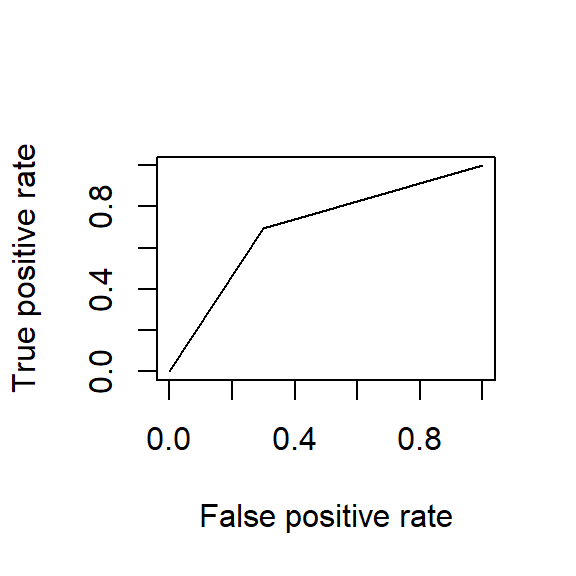auc <- performance(pr, measure = "auc") auc <- auc@y.values[] auc ##  0.6997144 proflogis <- glm(credit.default ~ profession, df, family=binomial(link="logit")) summary(proflogis) ## ## Call: ## glm(formula = credit.default ~ profession, family = binomial(link = "logit"), ## data = df) ## ## Deviance Residuals: ## Min 1Q Median 3Q Max ## -1.7941 -0.7710 -0.6231 1.0508 1.8626 ## ## Coefficients: ## Estimate Std. Error z value Pr(>|z|) ## (Intercept) -1.5404 0.6362 -2.421 0.01547 * ## professionworker 0.4796 0.7445 0.644 0.51950 ## professionteacher 1.8458 0.7272 2.538 0.01114 * ## professionself-employed 2.9267 0.9063 3.229 0.00124 ** ## --- ## Signif. codes: 0 '***' 0.001 '**' 0.01 '*' 0.05 '.' 0.1 ' ' 1 ## ## (Dispersion parameter for binomial family taken to be 1) ## ## Null deviance: 136.66 on 99 degrees of freedom ## Residual deviance: 115.75 on 96 degrees of freedom ## AIC: 123.75 ## ## Number of Fisher Scoring iterations: 4 auc(df$credit.default, predict(proflogis, type="response"))
## Area under the curve: 0.7438
pr <- prediction(predict(proflogis, type="response"), df$credit.default) prf <- performance(pr, measure = "tpr", x.measure = "fpr") plot(prf)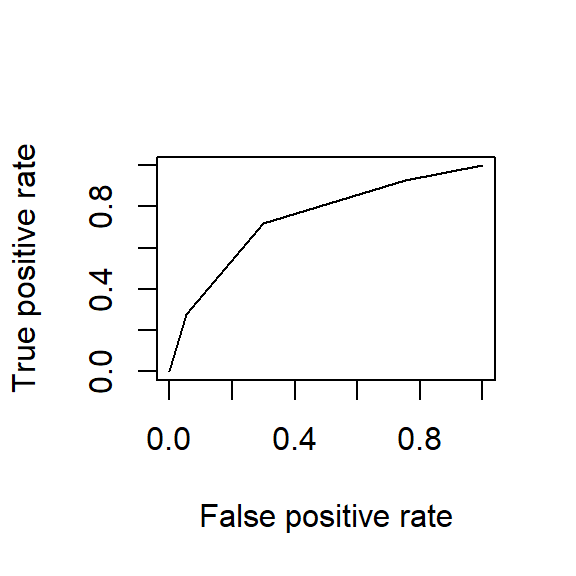auc <- performance(pr, measure = "auc") auc <- auc@y.values[] auc ##  0.743778 Another example from this video: set.seed(420) n.samples <- 100 weight <- sort(rnorm(n.samples,172,29)) obese <- ifelse(runif(n.samples) < (rank(weight)/100), yes=1, no=0) plot(weight,obese, pch=19, col='tan4', cex=.7) glm.fit <- glm(obese ~ weight, family="binomial") lines(weight, glm.fit$fitted.values)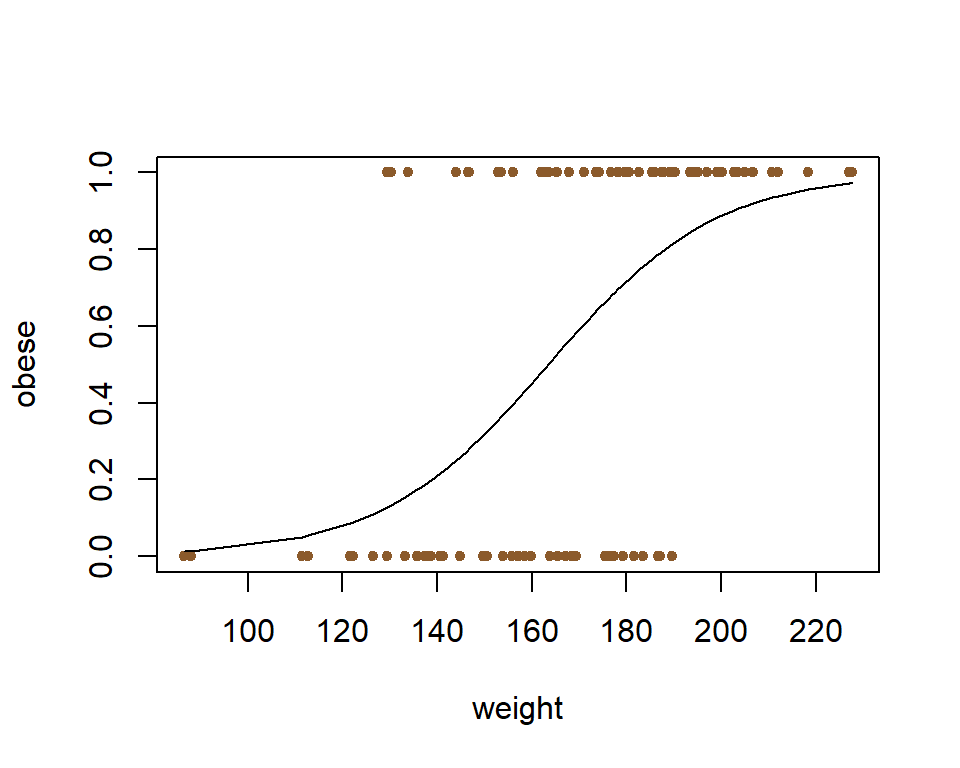par(pty="s")
roc(obese, glm.fit$fitted.values, plot=T)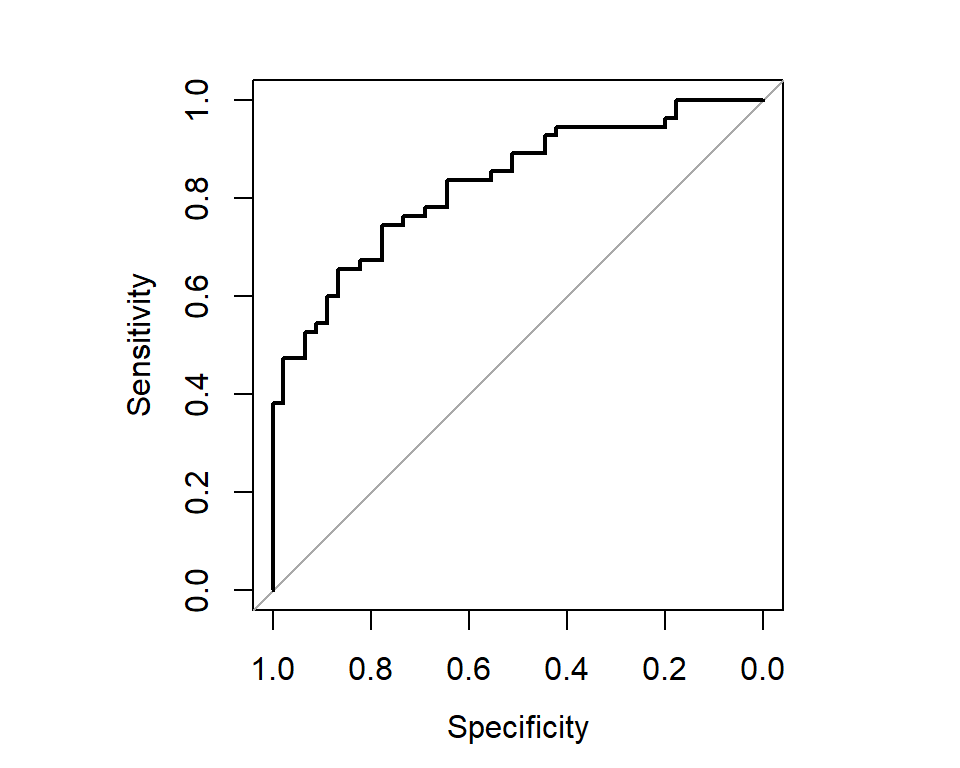## ## Call: ## roc.default(response = obese, predictor = glm.fit$fitted.values,     plot = T)
##
## Data: glm.fit$fitted.values in 45 controls (obese 0) < 55 cases (obese 1). ## Area under the curve: 0.8291 roc.info <- roc(obese, glm.fit$fitted.values)
roc.df <- data.frame(ttp=roc.info$sensitivities*100, fpp=(1-roc.info$specificities) *100, thresholds=roc.info$thresholds) head(roc.df) ## ttp fpp thresholds ## 1 100 100.00000 -Inf ## 2 100 97.77778 0.01349011 ## 3 100 95.55556 0.03245008 ## 4 100 93.33333 0.05250145 ## 5 100 91.11111 0.07017225 ## 6 100 88.88889 0.08798755 tail(roc.df) ## ttp fpp thresholds ## 96 9.090909 0 0.9275222 ## 97 7.272727 0 0.9371857 ## 98 5.454545 0 0.9480358 ## 99 3.636364 0 0.9648800 ## 100 1.818182 0 0.9735257 ## 101 0.000000 0 Inf Notice that the first row of head(roc.df) with a threshold for labeling “obese” of $$-\inf$$ the ttp (true positive percentage) will be of $$100\%,$$ and so will be the fpp (false positive percentage), and it corresponds to the upper-right hand part of the ROC curve. The bottom-left part of the curve corresponds to the last row of tail(roc.df). We can isolate part of the data, for example with ttp between $$60$$ and $$80$$, to select an optimal threshold value: roc.df[roc.df$ttp > 60 & roc.df$ttp < 80,] ## ttp fpp thresholds ## 42 78.18182 35.55556 0.5049310 ## 43 78.18182 33.33333 0.5067116 ## 44 78.18182 31.11111 0.5166680 ## 45 76.36364 31.11111 0.5287933 ## 46 76.36364 28.88889 0.5429351 ## 47 76.36364 26.66667 0.5589494 ## 48 74.54545 26.66667 0.5676342 ## 49 74.54545 24.44444 0.5776086 ## 50 74.54545 22.22222 0.5946054 ## 51 72.72727 22.22222 0.6227449 ## 52 70.90909 22.22222 0.6398136 ## 53 69.09091 22.22222 0.6441654 ## 54 67.27273 22.22222 0.6556705 ## 55 67.27273 20.00000 0.6683618 ## 56 67.27273 17.77778 0.6767661 ## 57 65.45455 17.77778 0.6802060 ## 58 65.45455 15.55556 0.6831936 ## 59 65.45455 13.33333 0.6917225 ## 60 63.63636 13.33333 0.6975300 ## 61 61.81818 13.33333 0.6982807 We can plot the ROC differently: par(pty="s") roc(obese, glm.fit$fitted.values, plot=T, legacy.axes=T, percent=T,
xlab='False positive percentage', ylab='True positive percentage',
col="darkslateblue",lwd=4, print.auc=T)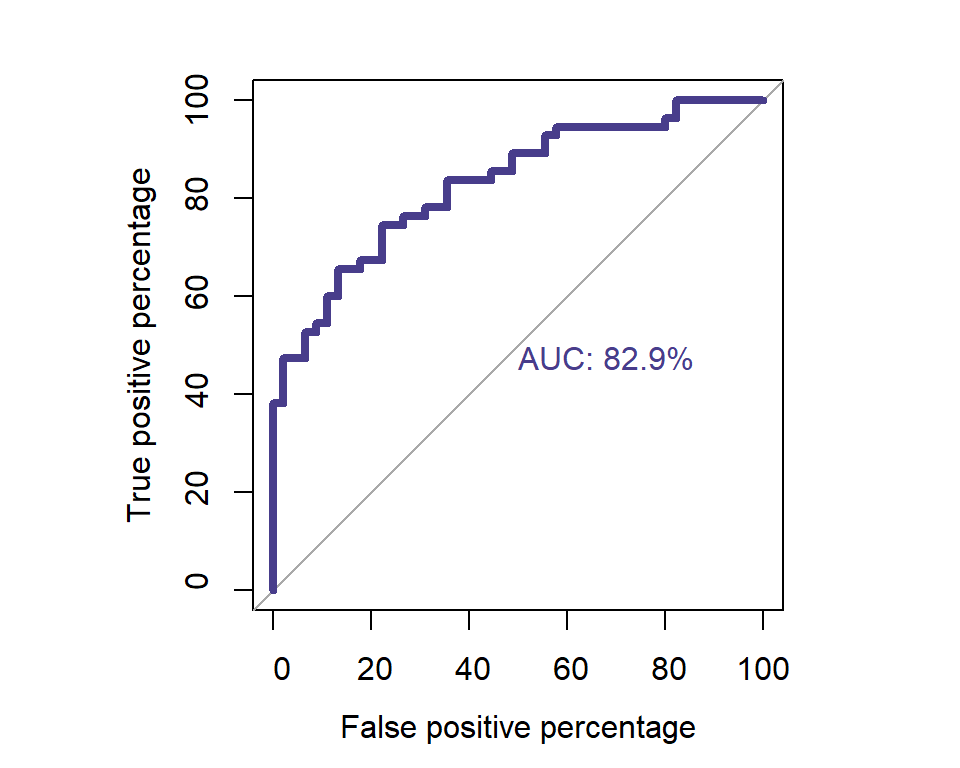##
## Call:
## roc.default(response = obese, predictor = glm.fit$fitted.values, percent = T, plot = T, legacy.axes = T, xlab = "False positive percentage", ylab = "True positive percentage", col = "darkslateblue", lwd = 4, print.auc = T) ## ## Data: glm.fit$fitted.values in 45 controls (obese 0) < 55 cases (obese 1).
## Area under the curve: 82.91%

To calculate the partial AUC of the beginning, where only a small number of false positives are allowed…

par(pty="s")
roc(obese, glm.fit$fitted.values, plot=T, legacy.axes=T, percent=T, xlab='False positive percentage', ylab='True positive percentage', col='darkslateblue',lwd=4, print.auc=T, print.auc.x=45, partial.auc=c(100,90), auc.polygon=T, auc.polygon.col='midnightblue')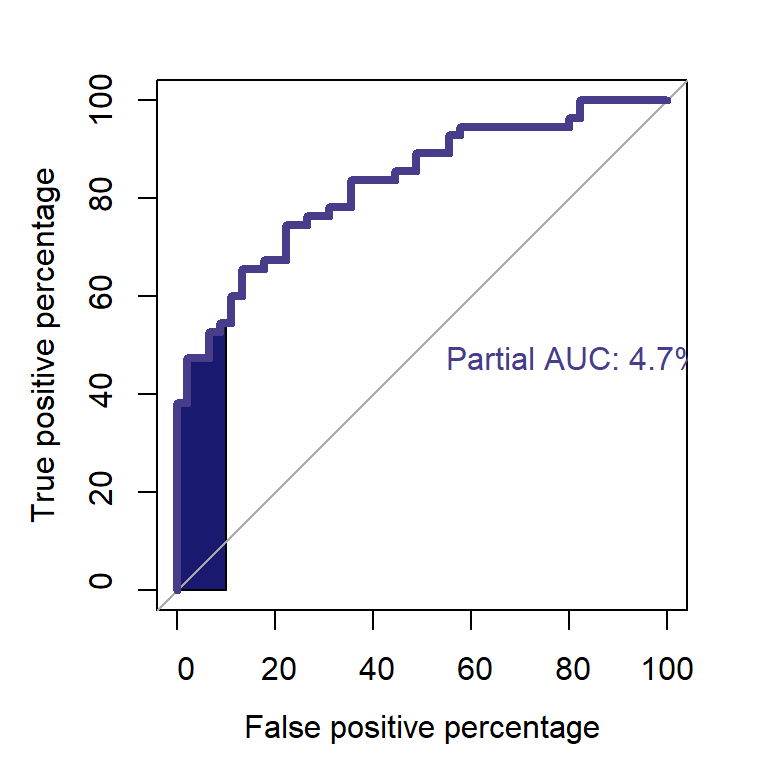## ## Call: ## roc.default(response = obese, predictor = glm.fit$fitted.values,     percent = T, plot = T, legacy.axes = T, xlab = "False positive percentage",     ylab = "True positive percentage", col = "darkslateblue",     lwd = 4, print.auc = T, print.auc.x = 45, partial.auc = c(100,         90), auc.polygon = T, auc.polygon.col = "midnightblue")
##
## Data: glm.fit$fitted.values in 45 controls (obese 0) < 55 cases (obese 1). ## Partial area under the curve (specificity 100%-90%): 4.727% Can we bootstrap? ci.auc(roc(obese, glm.fit$fitted.values), conf.level=0.95, method=
"bootstrap", boot.n = 2000, boot.stratified = TRUE, reuse.auc=TRUE,
progress = getOption("pROCProgress")\$name, parallel=FALSE)
## 95% CI: 0.7471-0.9006 (2000 stratified bootstrap replicates)

####TESTING A LOGISTIC REGRESSION MODEL:

1. GOODNESS-OF-FIT: Model calibration

The Hosmer-Lemeshow goodness of fit test divides up in boxes the predicted probabilities (in R the function is fitted as opposed to predict), and runs a chi-square test comparing to the percentage of cases that have $$Y=1$$ among those with predicted probability within a certain interval. See here:

The Hosmer-Lemeshow goodness of fit test is based on dividing the sample up according to their predicted probabilities, or risks. Specifically, based on the estimated parameter values $$\hat{\beta}_{0},\hat{\beta}_{1},..,\hat{\beta}_{p},$$ for each observation in the sample the probability that $$Y=1$$ is calculated, based on each observation’s covariate values:

$\hat{\pi} = \frac{\exp(\hat{\beta}_{0}+\hat{\beta}_{1}X_{1}+..+\hat{\beta}_{p}X_{p})}{1+\exp(\hat{\beta}_{0}+\hat{\beta}_{1}X_{1}+..+\hat{\beta}_{p}X_{p})}$

The observations in the sample are then split into $$g$$ groups (we come back to choice of g later) according to their predicted probabilities. Suppose (as is commonly done) that $$g=10.$$ Then the first group consists of the observations with the lowest $$10\%$$ predicted probabilities. The second group consists of the $$10\%$$ of the sample whose predicted probabilities are next smallest, etc etc.

Suppose for the moment that all of the observations in the first group had a predicted probability of $$0.1.$$ Then, if our model is correctly specified, we would expect the proportion of these observations who have $$Y=1$$ to be $$10\%.$$ Of course, even if the model is correctly specified, the observed proportion will deviate to some extent from 10%, but not by too much. If the proportion of observations with $$Y=1$$ in the group were instead $$90\%,$$ this is suggestive that our model is not accurately predicting probability (risk), i.e. an indication that our model is not fitting the data well.

In practice, as soon as some of our model covariates are continuous, each observation will have a different predicted probability, and so the predicted probabilities will vary in each of the groups we have formed. To calculate how many Y=1 observations we would expect, the Hosmer-Lemeshow test takes the average of the predicted probabilities in the group, and multiplies this by the number of observations in the group. The test also performs the same calculation for $$Y=0,$$ and then calculates a Pearson goodness of fit statistic

$\sum^{1}_{k=0} \sum^{g}_{l=1} \frac{(o_{kl}-e_{kl})^{2}}{e_{kl}}$

where $$o_{0l}$$ denotes the number of observed $$Y=0$$ observations in the $$l$$-th group, $$o_{1l}$$ denotes the number of observed $$Y=1$$ observations in the $$l$$-th group, and $$e_{0l}$$ and $$e_{1l}$$ similarly denote the expected number of zeros.

In a 1980 paper Hosmer-Lemeshow showed by simulation that (provided $$p+1<g$$) their test statistic approximately followed a chi-squared distribution on $$g-2$$ degrees of freedom, when the model is correctly specified. This means that given our fitted model, the p-value can be calculated as the right hand tail probability of the corresponding chi-squared distribution using the calculated test statistic. If the p-value is small, this is indicative of poor fit.

It should be emphasized that a large p-value does not mean the model fits well, since lack of evidence against a null hypothesis is not equivalent to evidence in favor of the alternative hypothesis. In particular, if our sample size is small, a high p-value from the test may simply be a consequence of the test having lower power to detect mis-specification, rather than being indicative of good fit.

1. C-STATISTIC or AUC (area under the curve of the ROC): Discriminatory power of the model

It is based on selecting different probabilities from $$0$$ to $$1$$ and calculating sensitivity and specificity in an ROC curve, and then measuring the AUC. See here:

Our model or prediction rule is perfect at classifying observations if it has 100% sensitivity and 100% specificity. Unfortunately in practice this is (usually) not attainable. So how can we summarize the discrimination ability of our logistic regression model? For each observation, our fitted model can be used to calculate the fitted probabilities $$P(Y=1|X_{1},..,X_{p}).$$ On their own, these don’t tell us how to classify observations as positive or negative. One way to create such a classification rule is to choose a cut-point $$c,$$ and classify those observations with a fitted probability above $$c$$ as positive and those at or below it as negative. For this particular cut-off, we can estimate the sensitivity by the proportion of observations with $$Y=1$$ which have a predicted probability above $$c,$$ and similarly we can estimate specificity by the proportion of $$Y=0$$ observations with a predicted probability at or below $$c.$$ If we increase the cut-point $$c,$$ fewer observations will be predicted as positive. This will mean that fewer of the $$Y=1$$ observations will be predicted as positive (reduced sensitivity), but more of the $$Y=0$$ observations will be predicted as negative (increased specificity). In picking the cut-point, there is thus an intrinsic trade off between sensitivity and specificity. Now we come to the ROC curve, which is simply a plot of the values of sensitivity against one minus specificity, as the value of the cut-point $$c$$ is increased from $$0$$ through to $$1.$$ Example:

set.seed(63126)
n <- 1000
x <- rnorm(n)
pr <- exp(x)/(1+exp(x))
y <- 1*(runif(n) < pr)
mod <- glm(y~x, family="binomial")
predpr <- predict(mod,type=c("response"))
library(pROC)
roccurve <- roc(y ~ predpr)
plot(roccurve)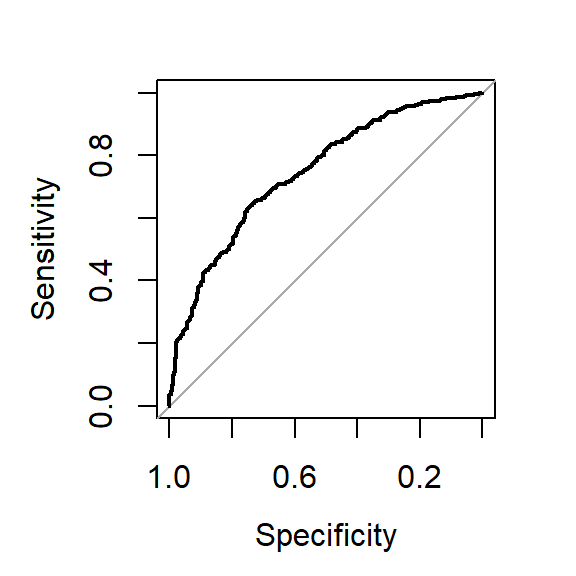A popular way of summarizing the discrimination ability of a model is to report the area under the ROC curve. We have seen that a model with discrimination ability has an ROC curve which goes closer to the top left hand corner of the plot, whereas a model with no discrimination ability has an ROC curve close to a $$45$$ degree line. Thus the area under the curve ranges from $$1,$$ corresponding to perfect discrimination, to $$0.5,$$ corresponding to a model with no discrimination ability. The area under the ROC curve is also sometimes referred to as the c-statistic (c for concordance).

auc(roccurve)
## Area under the curve: 0.7463

The ROC curve (AUC) has a somewhat appealing interpretation. It turns out that the AUC is the probability that if you were to take a random pair of observations, one with $$Y=1$$ and one with $$Y=0,$$ the observation with $$Y=1$$ has a higher predicted probability than the other. The AUC thus gives the probability that the model correctly ranks such pairs of observations.

In the biomedical context of risk prediction modelling, the AUC has been criticized by some. In the risk prediction context, individuals have their risk of developing (for example) coronary heart disease over the next 10 years predicted. Thus a measure of discrimination which examines the predicted probability of pairs of individuals, one with $$Y=1$$ and one with $$Y=0,$$ does not really match the prospective risk prediction setting, where we do not have such pairs.